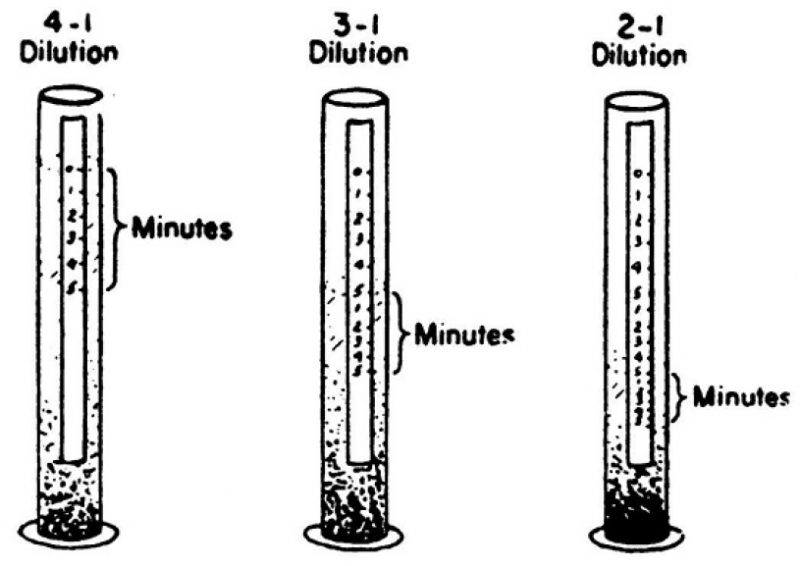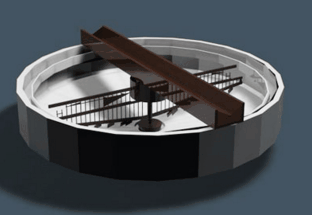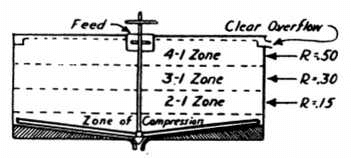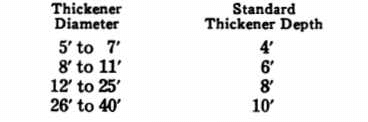# How to Size a Thickener

Knowing how to size a thickener and determine the capacity required to handle a pre-determined tonnage of a certain pulp, by overflowing a clear solution and obtaining the desired pulp density of thickener discharge, depends upon the settling rate of that particular pulp. The settling rate of any pulp is easily determined by simple laboratory tests such as outlined below:

Laboratory Test Method:

Place a measured quantity of pulp at a known density in a beaker or glass cylinder. Fix a narrow strip of paper on one side of the container. Mix pulp thoroughly. Draw a line on the paper at the top of the pulp and mark “0” minutes. For five minutes, at one-min­ute intervals, mark the point to which the solids have settled. This determines the free settling rate of the solids at the initial density.Usually readings should be taken at three different densities of the pulp corresponding approximately to densi­ties which will exist in the various zones in the thickener.

Decant sufficient clear water or solu­tion to establish a pulp with intermedi­ate density. For instance, if initial pulp density was 4:1, water to solids, the re­moval of one-fourth of the water would establish a density of 3:1. Mix thor­oughly. Repeat readings of settlement as above.

Then decant again to obtain a pulp at the third density. The pulp just tested was at 3:1 dilution, so decanting one- third of the water will give 2:1 dilution, water to solids. Mix thoroughly. Repeat settling measurements at one-minute intervals for five minutes.

The settling rate per minute should be uniform during the testing at each dilution, until compression is reached, at which time the amount of settling will decrease during each succeeding minute. Measure the settling marks in inches, thus determining the settling rate in inches per minute for each pulp density, and convert this to feet per hour.

Determining Final Density:

Final density is then determined thoroughly mix the pulp remaining after the test at 2:1 dilution and allow to settle for 19 hours. Mark the position of settled pulp and let stand for a few hours to see if final density was reached. If pulp continues to settle, mark its po­sition at hourly intervals until settling stops. Decant off all clear water or so­lution. Then determine moisture con­tent of pulp by weighing and drying.

Calculating Thickener Area:Thickener area required is then cal­culated by applying above determined data in the following formula:

A = 1.333 (F – D)/R

A = Thickener area in square feet per ton of dry solids thickened in 24 hours.
F = Initial density (Parts Water to Sol­ids by weight).
D = Final density to which pulp will settle or density at which you want to discharge pulp from thickener.
R = Settling rate in feet per hour.

Usually it is desired to discharge pulp from the thickener at its final density as shown in the above test. However, if you want to dis­charge pulp more diluted than the actual final density, the density desired should be used in above formula rather than the final density to which the pulp will settle.

Calculations of indicated thickener area from each of the three settling rates obtained in tests will indicate any change in settling rate in the different zones of the thickener, and the largest area obtained from the three calcula­tions should be used.

Assume the following data was ob­tained from the above tests:

At 4:1 dilution R = 0.50 feet per hour
At 3:1 dilution R = 0.30 feet per hour
At 2:1 dilution R = 0.15 feet per hour
Final Density D = 1:1Applying this data to above formula, you obtain:

A = 1.33 (4 – 1)/0.50 = 7.98

A = 1.33 (3 – 1)/0.30 = 8.86

A = 1.33 (2 – 1)/0.15 = 8.87

Factor of Safety:

Normally a 25 per cent factor of safety is allowed in determining the thickener area size. Thus, in the above case, 8.87 (largest of three figures) plus 25 per cent factor of safety, equals 11.09 square feet area required per ton of dry solids fed to the thickener per 24 hours.

If the pulp reached its final density during the 19 hour test, a standard depth thickener is considered adequate. However, if additional time was re­quired to reach final density, the thick­ener volume would have to be large enough to retain the pulp for this extra time.

Standard thickener depths are as follows:Storage Capacity:

Another factor to be considered is the storage capacity desired in a thickener. For example, it may be necessary to shut a filter down for repairs and at the same time keep the balance of the mill in operation. In this case the thick­ener will act as a storage reservoir. Where storage capacity in a thickener is of importance such as in many of the non-metallic flotation plants, a larger factor of safety should be applied in cal­culating the area. Greater depth should not be used unless the lift of the thick­ener mechanism can also be increased.

Duplicating Thickener Action:

Many times a pulp standing without agitation will not settle to as low a final density as when aided by movement of thickener rakes in actual operation our Laboratory developed a miniature thickener mechanism which is used in determining final density un­der actual operating conditions.

Pulp Calculations for CLASSIFIERS AND THICKENERS

Given percentage moisture (or solids, 100—Moisture) in feed, sands and overflow, and tonnage (dry) of feed sand or overflow.

Let F, S, and Q be tons (dry) of feed. Sands (spigot) and Overflow, respectively.
Let, f, s, and q be percent of Moisture in Feed, Sands and Overflow, respectively.
S+Q = F and F/100 – f = Q/100-q + S = 100-s
S = F 100-s/100-f x q-f/q-s
Q = F 100-q/100-f x f-s/q-s
F = Q 100-f/100-q x q-s/f-s = S 100 – f- (a-s)/100 – s-(a-f)
To determine content of any one pulp, the other two moisture contents must be known as well as all the tonnages.

EXAMPLE:—Tons solid feed per hour 150, Moisture feed 49%, sands 31%, Overflow 82%.
F = 150, f = 49, s = 31, q = 82.
Sand Tonnage S = F 100-s/100-f x q – f/q -s = 150 100-31/100-49 x 82-49/82-31 = 150 69/51 x 33/51 = 131 tons sands.
Q = F 100-q/100-f x f-s/q-s = 150 100-82/100-49 x 49-31/82-31 = 150 81/51 x 18/51 = 18.7 tons overflow.
Total……………………………………………..149.7 tons O+S = Feed
Error 0.3 tons due to slight inaccuracies in original data.

Specific Gravity

DETERMINATIONS

Let W= weight of a given volume of water (1 gram = 1 cc.).
Ws = weight of an equal volume of dry solids with voids.
Wp= weight of an equal volume of pulp, or by wetting solids to make water level equal.
Gs = specific gravity of solids= Ws/W – (Wp-Ws)
Gp =specific gravity of pulp= Wp/W
Ws = Gp-1/Gs-1 x GsW
S=percent of solids in pulp=100 x Gp-1/Gs-1 x Gs/Gp
Weight per cubic foot = Gp/32.038 tons = 62.425 Gp pounds
Cubic Feet per ton=32.038/Gp
Cubic Feet pulp per ton dry solids=32.038 (Gs-1)/(Gp-1) Gs
Per cent water in pulp=100 -S=100 Gs-Gp/(Gs-1) Gp
Tons of dry solids per foot depth for round tanks of diameter.
D (in feet)= D² (Gp – 1) Gs/40.8 (Gs – 1)